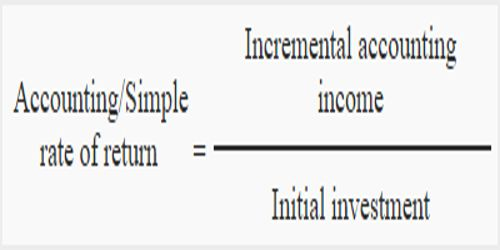# Accounting Rate of Return (ARR):

Accounting Rate of Return (ARR) is the amount of profit, or return, that an individual can expect based on an investment made. Accounting rate of return divides the average profit by the initial investment in order to get the ratio or return that can be expected. It is the ratio of estimated accounting profit of a project to the average investment made in the project. This allows an investor or business owner to easily compare the profit potential for projects, products, and investments. ARR does not consider the time value of money or cash flows, which can be an integral part of maintaining a business.

Accounting Rate of Return is calculated using the following formula:

ARR = [Average Accounting Profit / Average Investment]

If the ARR is equal to 5%, this means that the project is expected to earn five cents for every dollar invested per year. The result of the calculation is expressed as a percentage.